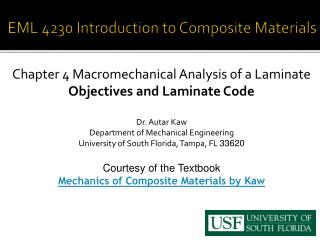Download PresentationEML 4230 Introduction to Composite Materials

# EML 4230 Introduction to Composite Materials

Download Presentation## EML 4230 Introduction to Composite Materials

- - - - - - - - - - - - - - - - - - - - - - - - - - - E N D - - - - - - - - - - - - - - - - - - - - - - - - - - -
##### Presentation Transcript

1. EML 4230 Introduction to Composite Materials Chapter 4 Macromechanical Analysis of a Laminate Objectives and Laminate Code Dr. Autar Kaw Department of Mechanical Engineering University of South Florida, Tampa, FL 33620 Courtesy of the Textbook Mechanics of Composite Materials by Kaw

2. Laminate Stacking Sequence

3. Chapter Objectives • Understand the code for laminate stacking sequence • Develop relationships of mechanical and hygrothermal loads applied to a laminate to strains and stresses in each lamina • Find the elastic stiffnesses of laminate based on the elastic moduli of individual laminas and the stacking sequence • Find the coefficients of thermal and moisture expansion of a laminate based on elastic moduli, coefficients of thermal and moisture expansion of individual laminas, and stacking sequence

4. Laminate Code

5. Laminate Code

6. Laminate Code

7. Laminate Code

8. Laminate Code

9. Special Types of Laminates • Symmetric Laminate: For every ply above the laminate midplane, there is an identical ply (material and orientation) an equal distance below the midplane. • Balanced Laminate: For every ply at a + orientation, there is another ply at the – orientation somewhere in the laminate.

10. Special Types of Laminates • Cross-ply Laminate: Composed of plies of either or (no other ply orientation). • Quasi-isotropic laminate: Produced using at least three different ply orientations, all with equal angles between them. Exhibits isotropic extensional stiffness properties.

11. Laminate Behavior • elastic moduli • the stacking position • thickness • angles of orientation • coefficients of thermal expansion • coefficients of moisture expansion

12. Strains in a beam (4.1)

13. Types of loads allowed in CLT analysis Nx = normal force resultant in the x direction (per unit length) Ny = normal force resultant in the y direction (per unit length) Nxy = shear force resultant (per unit length)

14. Types of loads allowed in CLT analysis Mx = bending moment resultant in the yz plane (per unit length) My = bending moment resultant in the xz plane (per unit length) Mxy = twisting moment resultant (per unit length)

15. END